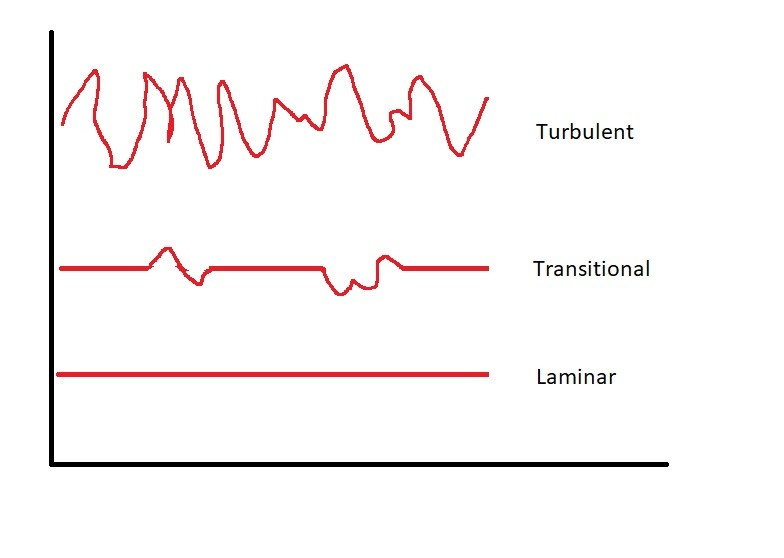# Introduction to Laminar and Turbulent Flow

As fluid flows through a pipe the flow could be laminar, turbulent, or in transition.  Laminar flow and turbulent flow was discovered by Osborn Reynolds.  During a set of experiments, Reynolds injected a dye into a fluid moving through a pipe.  The pipe would have some diameter $D$ while the fluid was moving at a velocity $v$.  For flows that had a “small enough” flowrate the dye would form a well defined line or streak.  As a result, a streakline would form.  Than as the flow increased there would be bursts of irregular behavior along the streakline.  Finally, the streakline would eventually become blurred and unrecognizable.  This in turn describes laminar, transitional, and turbulent flow.The reason why dye cannot be used to observe turbulent flow is because the random fluctuations will disperse the die throughout the fluid.   On the other hand, laminar flow moves at a constant velocity allowing a streakline to form.  In turn, laminar flow will only have one component of velocity which will be in the direction of the flow. As seen in the following mathematical expression.

(Eq 1) $v=u\hat{i}$

However, the velocity for turbulent flow is dictated by random unsteady flow.  As a result, the velocity isn’t necessarily going in the direction of the flow.  The following mathematical expression is needed to find the velocity of fluid particle in turbulent flow.

(Eq 2) $v=u\hat{i}+ν\hat{j}+w\hat{k}$

In addition, since turbulent flow is random, the position of particles will differ at any given time which is not easily predicted.

### Laminar and Turbulent Flow and Reynolds Number

To correctly determine whether a flow is laminar or turbulent Reynolds Number will need to be used. To find Reynolds number the following equation will be used.

(Eq 3) $Re=\frac{ρvD}{μ}$

$ρ$ = fluid density

$v$ =  average fluid velocity

$D$ = pipe diameter

$μ$ = dynamics viscosity

For flows in a round pipe, if the Reynolds Number is less than 2100 the flow is considered turbulent.  On the other hand, if Reynolds Number is great than 4000 the flow is turbulent.  In addition, anything in between those two number is in transition from laminar to turbulent flow.  However, these numbers are only a baseline.  Different surface roughness of the pipe wall and if there is any vibration in the pipe will affect the transition from laminar to turbulent flow.

|